### 东风日产 楼兰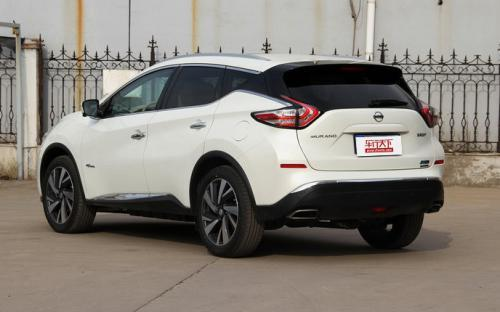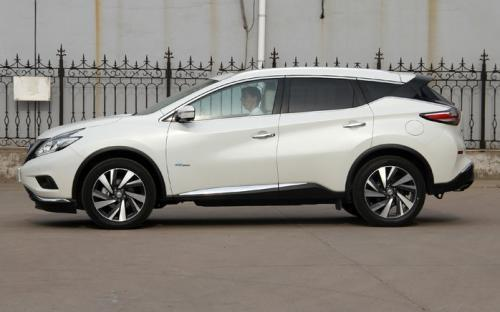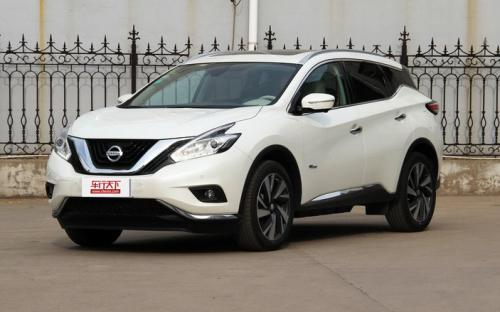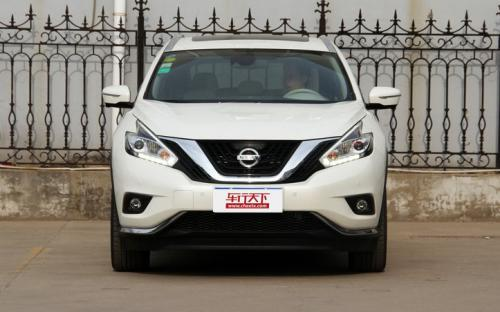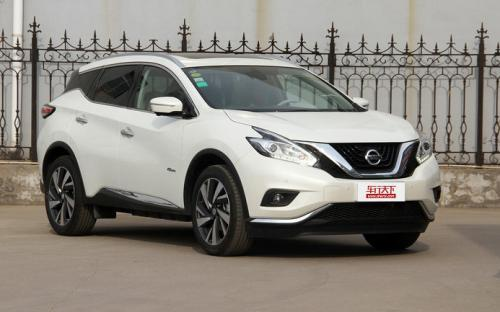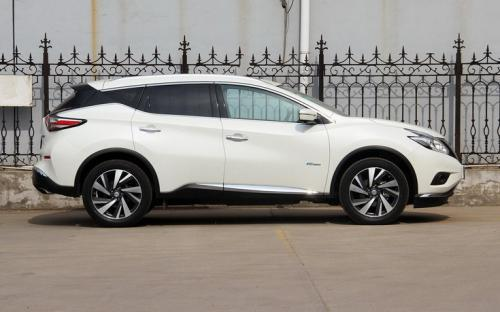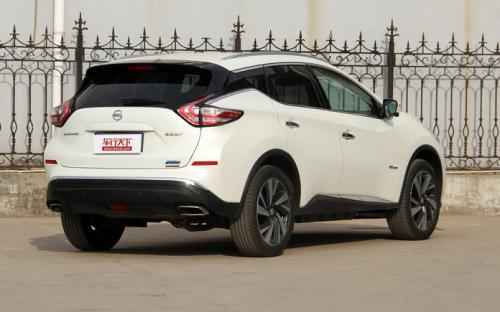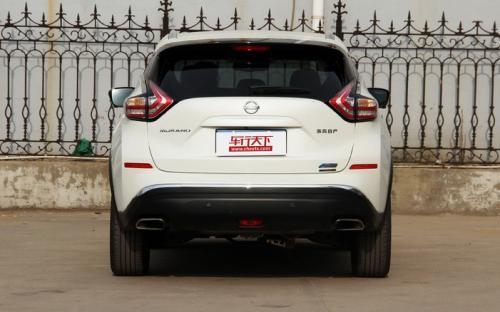6 种颜色可选2016款最低售价：31.28 万元起

---##### 配置亮点：
• 胎压监测装置

• ISOFIX儿童座椅接口

• 车身稳定控制(ESC/ESP/DSC等)

• 电动天窗

• 定速巡航

• 后倒车雷达

• 真皮座椅

• GPS导航系统

• 氙气大灯

• 后视镜加热

• 提交
2016款 2.5 S/C HEV XL 四驱混动尊贵版 (150张)

东风日产 楼兰 绕车实拍• 东风日产 楼兰 在售车型

排量 车型 厂商指导价 本地最低报价 购车工具
2.5L
2.5 S/C HEV XL 两驱混动尊锐版 油电混合 CVT无级变速(模拟7挡)
31.28万
31.28万

2.5 S/C HEV XL 四驱混动尊贵版 油电混合 CVT无级变速(模拟7挡)
33.38万
33.38万

东风日产 楼兰 经销商

查看更多 >>

### 东风日产 楼兰 动力加速

楼兰 0-100公里加速时间分布在 9.1-9.5秒 属于 民用级

动力级别 加速时间 车型
民用级(2款)
9.1s
2.5 S/C HEV XL 两驱混动尊锐版
9.5s
2.5 S/C HEV XL 四驱混动尊贵版

东风日产 楼兰 视频

东风日产 楼兰 新闻资讯

# 日产楼兰新车型上市 售价31.28-33.38万元

上市新车 超过8317次关注

东风日产宣布楼兰新增两款混动车型正式上市，新车在外观方面与在售的楼兰保持一致，配置增加，其售价区间为31.28-33.38万元。

# 中高配置SUV对比 别克昂科威VS日产楼兰

评测 超过9402次关注

如今中型SUV市场的竞争向来十分激烈，搭载2.5L自然吸气发动机的大多数车型指导价都已下探至30万元以内，对于那些并不是十分追求动力的消费者来说，可谓是不错的选择。

# 深度试驾东风日产楼兰2.5T四驱混动

评测 超过11194次关注

日产楼兰有着动感的外形，助力略沉的方向盘却还保留了不错的路感。对于消费者来说最有吸引力的自然是它的定价，入门级相比老款低了10余万的售价加上丰富的配置，新...

# 售23.88-37.98万 日产全新楼兰正式上市

新闻 超过9636次关注

8月8日，东风日产新一代楼兰在上海正式上市，新车共推9款车型，含两款2.5L自吸车型以及两款2.5T混动版本，售价区间为23.88-37.98万。

# 颠覆感官 试驾SUV新楼兰

试驾评测 超过9337次关注

在海南万宁举行的“独创一帜领潮而来”东风日产新楼兰全国媒体试驾活动中，新楼兰在造型、动力、豪华科技配置以及主被动安全方面，的确具有颠覆性的产品优势，一改...

# 试驾东风日产楼兰 沉迷在那温柔乡

试驾评测 超过3487次关注

带着一身寒意坐进楼兰，启动车辆，打开座椅加热，不消片刻温暖的感觉便开始从后背和腿部涌向全身。走不上两三公里，暖风系统已能正常工作，身体随之从僵硬中苏醒。...

# 试驾日产楼兰Murano 没什么能阻挡

试驾评测 超过3245次关注

东风日产将Murano命名为“楼兰”真是一件不可思议的事情，居然用中国地名命名新车。事实上，Murano与中国西北沙漠中的楼兰古城半点关系都没有，它是意大利威尼斯城...

# 豪华车年底加速裂分 SUV等个性车型成新宠

车系文章 超过2244次关注

越豪华越好卖，这一车市“定律”在刚刚结束的广州车展上被演绎到极点。车展开幕仅两天就售出12辆价值百万以上的豪华车。而在车展前后，豪华车推出新品的力度更是远...

# 楼兰年内将推2.5L车型 意在降低身价

车系文章 超过3135次关注

日前从相关媒体处获悉，东风日产楼兰预计在今年下半年推出2.5L车型，意在通过降低入门价来获取更高的市场份额。

# 2014年投产 东风日产大连基地月底奠基

车系文章 超过2725次关注

日前，有可靠消息人士称，大连基地将在本月举行奠基仪式，正式开始建设东风日产的第四座生产基地，“目前已在协调政府等重要人士的出席时间，初定在本月25日。”

# 楼兰Murano少量现车 购车送3000元精品

车系文章 超过3063次关注

近日，编辑从东风日产郑州中原专营店了解到，楼兰Murano店内有少量现车供应，目前购车暂无任何优惠，购车可获赠3000元精品，感兴趣的朋友可以到4S店内进行了解。

猜你喜欢

﻿
• 快速找车
• 选择品牌
• 选择品牌
• A  奥迪
• A  阿斯顿·马丁
• A  阿尔法·罗密欧
• B  宝沃
• B  布加迪
• B  巴博斯
• B  保时捷
• B  宾利
• B  奔驰
• B  宝马
• B  本田
• B  别克
• B  标致
• B  比亚迪
• B  宝骏
• B  北汽制造
• B  北汽新能源
• B  北汽幻速
• B  北汽威旺
• B  北京汽车
• B  奔腾
• B  北汽绅宝
• C  长安
• C  长安商用
• C  长城
• C  昌河
• D  大众
• D  道奇
• D  DS
• D  东南
• D  东风风神
• D  东风风行
• D  东风小康
• D  东风风度
• D  东风
• F  福特
• F  丰田
• F  菲亚特
• F  法拉利
• F  福田
• F  福迪
• F  福汽启腾
• G  观致
• G  广汽传祺
• G  广汽吉奥
• G  GMC
• H  红旗
• H  汉腾汽车
• H  哈弗
• H  哈飞
• H  海格
• H  海马
• H  华颂
• H  黄海
• H  华泰
• H  恒天
• J  吉利汽车
• J  捷豹
• J  Jeep
• J  江淮
• J  江铃
• J  金杯
• J  九龙
• J  金旅
• K  凯翼
• K  凯迪拉克
• K  克莱斯勒
• K  科尼塞克
• K  卡威
• K  开瑞
• L  路虎
• L  林肯
• L  劳斯莱斯
• L  兰博基尼
• L  雷克萨斯
• L  铃木
• L  雷诺
• L  理念
• L  力帆
• L  莲花汽车
• L  猎豹
• L  路特斯
• L  陆风
• M  马自达
• M  MG
• M  MINI
• M  玛莎拉蒂
• M  摩根
• M  迈凯轮
• N  纳智捷
• O  欧宝
• O  讴歌
• O  欧朗
• Q  奇瑞
• Q  起亚
• Q  启辰
• R  日产
• R  荣威
• R  瑞麒
• S  三菱
• S  斯威汽车
• S  萨博
• S  smart
• S  斯柯达
• S  斯巴鲁
• S  思铭
• S  双龙
• S  上汽大通
• S  双环
• T  特斯拉
• T  腾势
• W  沃尔沃
• W  五菱汽车
• W  五十铃
• W  威兹曼
• W  威麟
• X  现代
• X  雪佛兰
• X  雪铁龙
• X  西雅特
• Y  一汽
• Y  英菲尼迪
• Y  英致
• Y  依维柯
• Y  野马汽车
• Y  永源
• Z  众泰
• Z  中华
• Z  中兴
• Z  知豆
• 选择车系
• 选择车系
• 车型对比
• 选择品牌
• 选择品牌
• A  奥迪
• A  阿斯顿·马丁
• A  阿尔法·罗密欧
• B  宝沃
• B  布加迪
• B  巴博斯
• B  保时捷
• B  宾利
• B  奔驰
• B  宝马
• B  本田
• B  别克
• B  标致
• B  比亚迪
• B  宝骏
• B  北汽制造
• B  北汽新能源
• B  北汽幻速
• B  北汽威旺
• B  北京汽车
• B  奔腾
• B  北汽绅宝
• C  长安
• C  长安商用
• C  长城
• C  昌河
• D  大众
• D  道奇
• D  DS
• D  东南
• D  东风风神
• D  东风风行
• D  东风小康
• D  东风风度
• D  东风
• F  福特
• F  丰田
• F  菲亚特
• F  法拉利
• F  福田
• F  福迪
• F  福汽启腾
• G  观致
• G  广汽传祺
• G  广汽吉奥
• G  GMC
• H  红旗
• H  汉腾汽车
• H  哈弗
• H  哈飞
• H  海格
• H  海马
• H  华颂
• H  黄海
• H  华泰
• H  恒天
• J  吉利汽车
• J  捷豹
• J  Jeep
• J  江淮
• J  江铃
• J  金杯
• J  九龙
• J  金旅
• K  凯翼
• K  凯迪拉克
• K  克莱斯勒
• K  科尼塞克
• K  卡威
• K  开瑞
• L  路虎
• L  林肯
• L  劳斯莱斯
• L  兰博基尼
• L  雷克萨斯
• L  铃木
• L  雷诺
• L  理念
• L  力帆
• L  莲花汽车
• L  猎豹
• L  路特斯
• L  陆风
• M  马自达
• M  MG
• M  MINI
• M  玛莎拉蒂
• M  摩根
• M  迈凯轮
• N  纳智捷
• O  欧宝
• O  讴歌
• O  欧朗
• Q  奇瑞
• Q  起亚
• Q  启辰
• R  日产
• R  荣威
• R  瑞麒
• S  三菱
• S  斯威汽车
• S  萨博
• S  smart
• S  斯柯达
• S  斯巴鲁
• S  思铭
• S  双龙
• S  上汽大通
• S  双环
• T  特斯拉
• T  腾势
• W  沃尔沃
• W  五菱汽车
• W  五十铃
• W  威兹曼
• W  威麟
• X  现代
• X  雪佛兰
• X  雪铁龙
• X  西雅特
• Y  一汽
• Y  英菲尼迪
• Y  英致
• Y  依维柯
• Y  野马汽车
• Y  永源
• Z  众泰
• Z  中华
• Z  中兴
• Z  知豆
• 选择车系
• 选择车系
• 选择车型
• 选择车型
• 意见反馈